# The product 2

The product of two functions is 10. If one of them is 2 1/3, find the other one.

b =  4.2857

### Step-by-step explanation: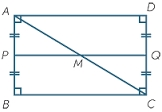Did you find an error or inaccuracy? Feel free to write us. Thank you!Tips to related online calculators
Need help to calculate sum, simplify or multiply fractions? Try our fraction calculator.
Do you have a linear equation or system of equations and looking for its solution? Or do you have a quadratic equation?

## Related math problems and questions:

• The product 3The product of two numbers is 15 ⁵/₆. If one of the numbers is 5 ²/₃, find the other.
• Two numbers and its productThe product of two numbers are 2/3. If on of them is 1/10, what is the other?
• Product increased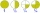When the product of 2/3 and 6/10 is increased by 2/5, the result is?
• The length 6The length of 12 pipes is 10 1/2 meters. (1) find the length of one pipe (2) also find the length of 7 pipes
• Evaluate fractionsThe difference  of 5 1/2 and 2/3 is added to the product of 5/6 and 1/2
• Divide 11Divide the product of 4 and 5/8 by 1 1/2. Write your answer as a mixed number.
• Without 2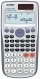Without multipying, tell whether the product 0.644 x 0.25 will be greater than 1 or less than 1? Explane how you know. Then find the product.
• Fraction equationEx over four is equal to two and one-fifth times ex plus three over ten. What is ex>
• What is 9What is the value of x in the proportion 2 and one-fourth over x = 1 and one-half over 3 and three-fifths? 2 and two-fifths 5 and two-fifths 8 and 1 over 10 12 and 3 over 20
• Product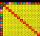The sum and the product of three integers are 6. Write largest of them.
• Fractions and mixed numerals(a) Convert the following mixed numbers to improper fractions. i. 3 5/8 ii. 7 7/6 (b) Convert the following improper fraction to mixed number. i. 13/4 ii. 78/5 (c) Simplify these fractions to their lowest terms. i. 36/42 ii. 27/45 2. evaluate following ex
• Quotient and productIf the quotient of [8/5 divided by 8/10] is added to the product of [8/14 x 7/12 x 3/8], what is the sum?
• ProductResult of the product of the numbers 1, 2, 3, 1, 2, 0 is:
• Simplify expression with mixedWhen (7¹/3 + 2¾)÷(2 + 2¼ × 1¹/3) is simplified the result is?
• Prime divisorsFind 2/3 of the sum's ratio and the product of all prime divisors of the number 120.
• There 11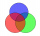There are 50 pupil in the class. Out of of this number,1/10 speak French only and 4/5 of the 10 remainder speak both French and English . If the rest speak English only find the number of students who speak only English?
• The fence 2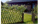A certain type of fencing sells for \$9 a yard. How much will 10 2/3 yards of fencing cost?